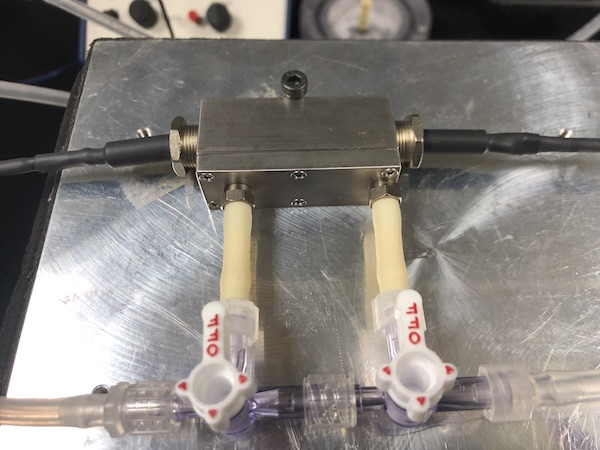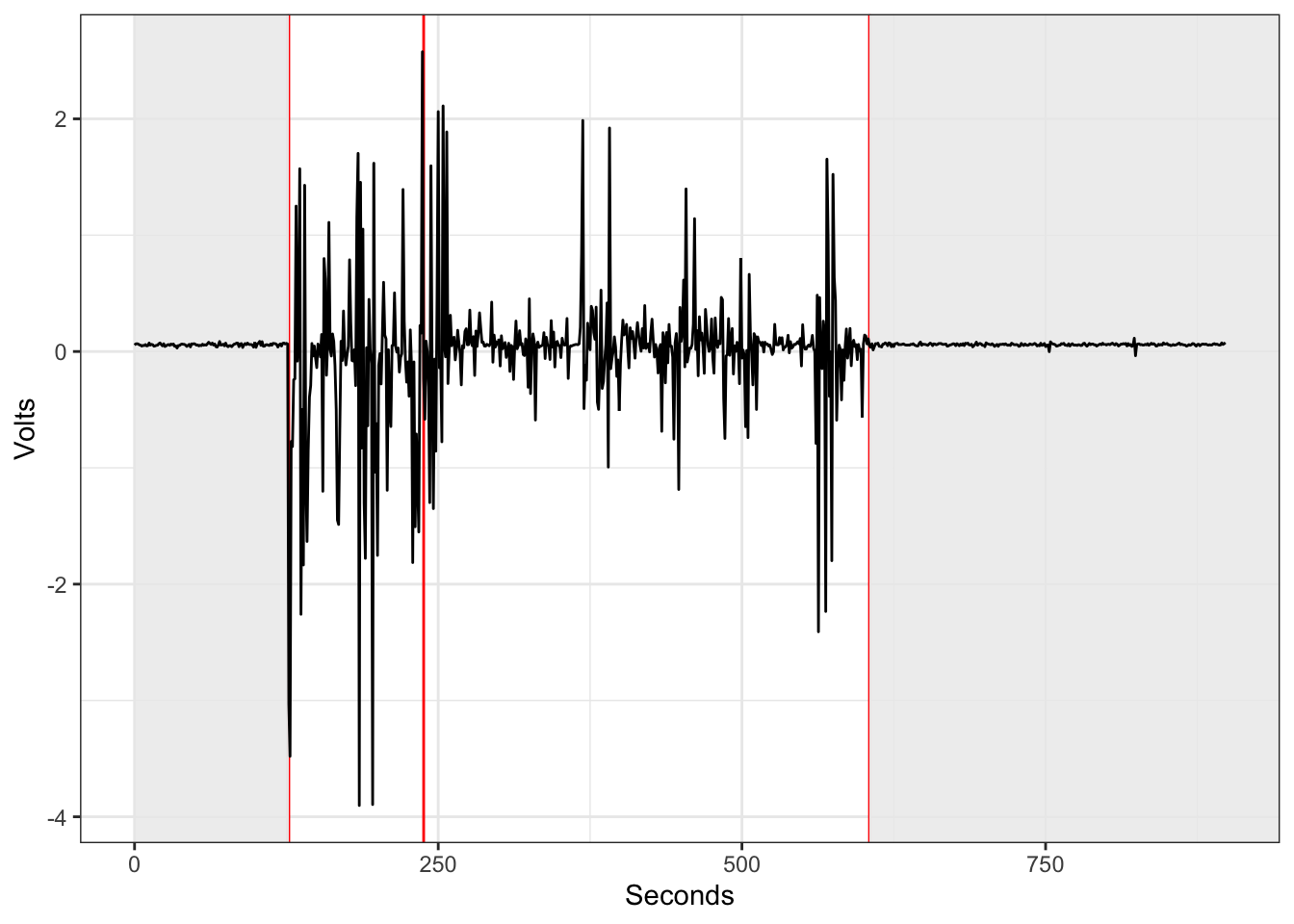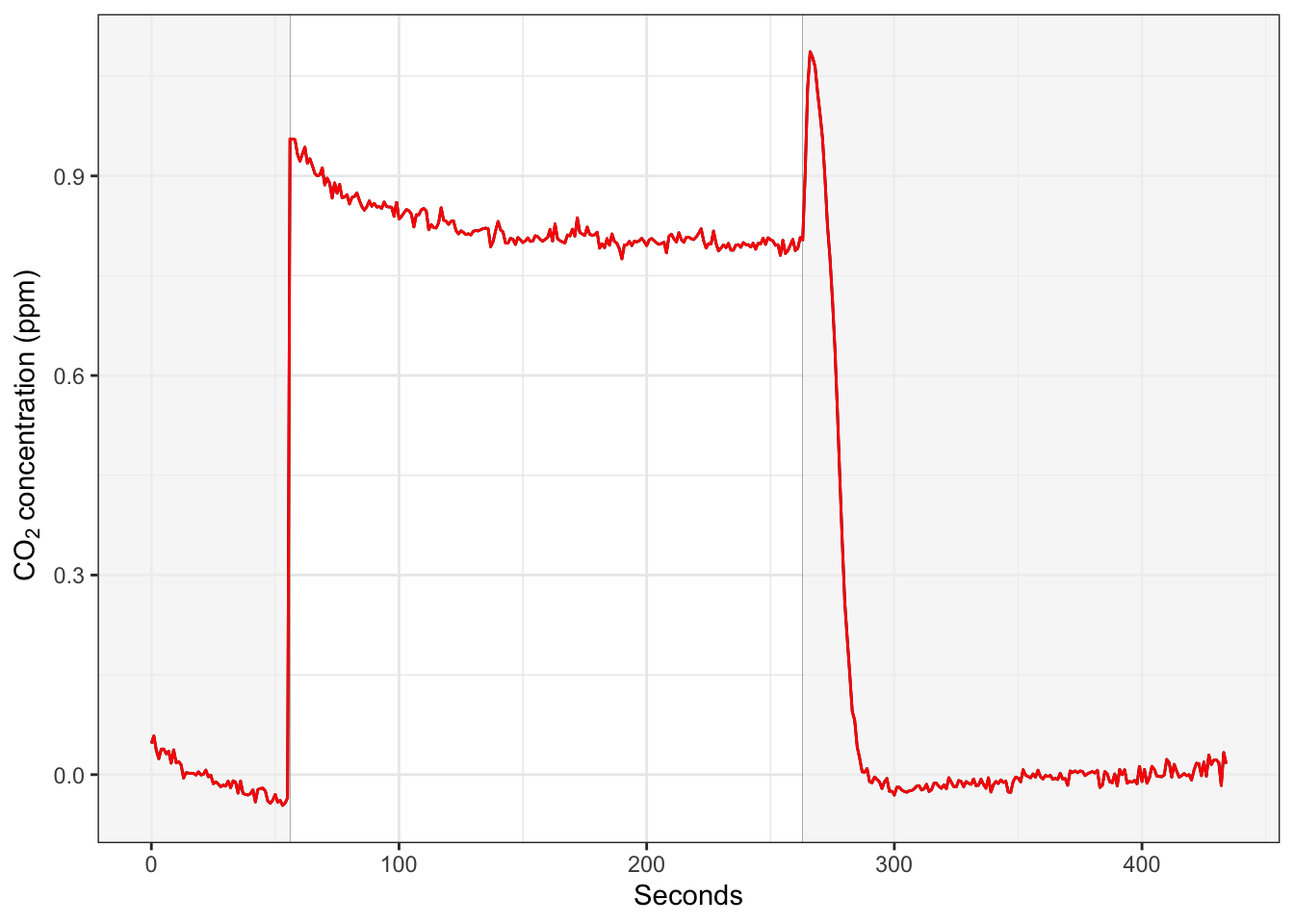Notes on setting up to do thermolimit respirometry.

#### New aluminum chambers

We had these respirometry chambers made by the ASU machine shop, based on the similar ones designed by Sable to use with their peltier-effect heating/cooling plate. The chambers are made from aluminum, have barbed fittings to attach tubing, there is a path through the top so that the incurrent air can thermally equilibrate, a cylindrical cavity to hold the insect, and two threaded caps which tighten onto an O-ring and which have glass windows to allow insertion of a pair of infrared emitter and detector.Aluminum chamber on pelt plate

With a pair of three-way valves, the respirometry airstream can be directed either into the chamber or diverted to flow around it (to offer a baseline).

Before using these, I had to reconfigure our old AD2, removing the static LED elements from the circuit board and attaching ones on cables so that they can be inserted into either end of the chamber. It worked!

#### Sample activity trace

``ggplot(dd, aes(x=Seconds, y=Volts))  + geom_vline(xintercept=c(127, 238, 605), col="red") + geom_rect(aes(ymin=-Inf, ymax=Inf, xmin=0, xmax=127), fill="grey95", alpha=0.1) + geom_rect(aes(ymin=-Inf, ymax=Inf, xmin=605, xmax=Inf), fill="grey95", alpha=0.1) + geom_path(size=0.5) + theme_bw()``Fruit fly activity (baseline - chamber - baseline)

#### Testing out the new respirometry chamber

I was measuring the metabolic rate of a tick and happened to realize that the the CO2 reading from the baseline air stream (0 ppm) did not match the CO2 reading from an empty aluminum chamber (~ 0.8 ppm).

This was puzzling because as far as I could tell, the chamber appeared well sealed and should’ve been airtight. Either something inside was producing CO2 or room air was diffusing into the chamber.

Taking a look at the data (note that I paused the recording for about a minute when switching between baseline/chamber paths to purge/washout any accumulated CO2):

``ggplot(c1, aes(x=Seconds, y=CO2_Li7k))  + geom_rect(aes(ymin=-Inf, ymax=Inf, xmin=-Inf, xmax=t1), fill="grey98", alpha=0.1) + geom_rect(aes(ymin=-Inf, ymax=Inf, xmin=t1, xmax=Inf), fill="grey98", alpha=.1) + geom_path(size=0.5) + geom_vline(xintercept=t1, col="grey50", size=0.1) + geom_path(size=0.5, col="red") + theme_bw() + ylab(bquote(~CO~'concentration (ppm)'))``Empty aluminum chamber

I checked a different kind of chamber (a simple tube design used with the MAVEn) and found that compared to the baseline, it only had a very slight elevation of 0.08 ppm while empty and in use, indicating a slight leak, but much smaller than with our new chambers.

``ggplot(c2, aes(x=Seconds, y=CO2_Li7k))  + geom_rect(aes(ymin=-Inf, ymax=Inf, xmin=-Inf, xmax=t2), fill="grey98", alpha=0.1) + geom_rect(aes(ymin=-Inf, ymax=Inf, xmin=t2, xmax=Inf), fill="grey98", alpha=.1) + geom_path(size=0.5) + geom_vline(xintercept=t2, col="grey50", size=0.1) + geom_path(size=0.5, col="red") + theme_bw() + ylab(bquote(~CO~'concentration (ppm)')) ``